In this step by step tutorials, you’ll learn how to use the INDEX function in Excel, with a clear syntax break-down and step by step examples demystified.

## What is INDEX function in Excel?

INDEX function retrieves a cell value from a range of cells, using the index numbers you specify.

The index function becomes very handy when you want to write a formula that finds a cell at the intersection of a specific row and column. This function recognizes the first row in the table as row one and the first column in the table as column 1.

Considering the following table, imagine you want to write a formula to find the intersection cell of row 7 and column 4 (i.e. the quantity of products bought by a customer named Jayden). You can solve this problem with the INDEX function.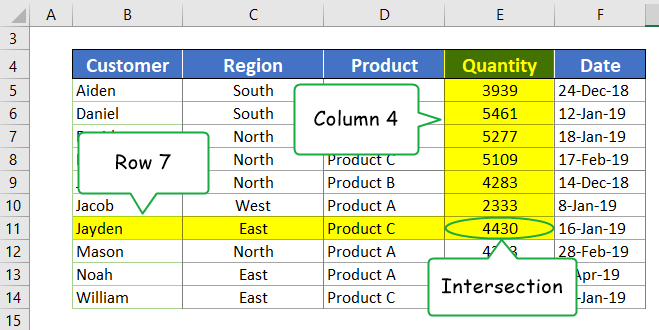This function works at its best when combined with other functions.

It is mostly used in conjunction with the MATCH function to perform lookups, which is much more flexible than both HLOOKUP and VLOOKUP function.

## The two forms of INDEX function

The index function comes in two forms, one is called the Array form and the other is called the Reference form.

Excel displays the syntax of both forms as soon as you type the function name with an opening bracket.

I’ll try and explain both versions in simple terms. I’ll begin with the first one which is the Array form.

It is worth noting that the array version of this function is used more often than the reference version, and hence you are also more likely to use it.INDEX function syntax (Array Form)

As I mentioned before, let’s understand how the array form of the function works before we look at the reference form. Below is the syntax breakdown of the Index function.

Syntax: INDEX(array, row_num, [column_num])This function has three arguments: array, row_num, and [column_num].

Before we get our hands on an example, lets quickly address these arguments one after the other. Knowing what a function argument does mean you are just halfway to mastering it.

So, below are the three arguments explained in simple terms.

• array: This represents a range of cells that has the data. This range could be a table or just an ordinary grid of cells. When specifying this range, make sure it covers all the rows and columns that contain your data.
• row_num: This represents a row number in a range. This row number is not referring to the row number in the actual worksheet. Rather, it is the relative row position in the table (range). This row number must not exceed the total number of rows in the table. When that happens, you’ll get a #REF! as a result which is Excel’s way of telling you that the cell you are referring to does not exist.
• [column_num]: This represents a column number in a range. This argument refers to the relative column position in the table (range) and not the actual worksheet column number. The column number must not be greater than the total number of columns in the table or else you’ll get #REF! as a result.

## What this function return

What this function return is a value found in the intersection cell of the row and column position specified.

Now let’s see how the INDEX function works in the following example.

### INDEX() function example 1 (Array form)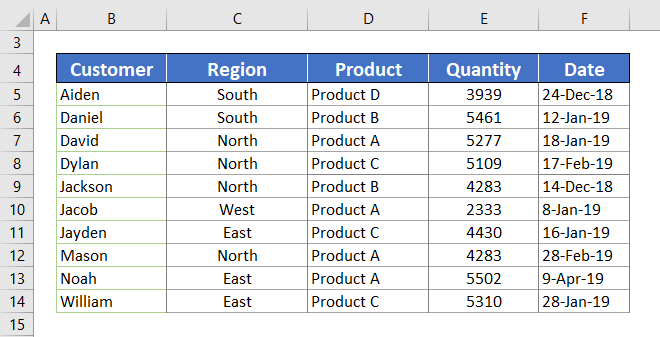Using the sample table above, let’s illustrate how the index function works. Assuming the above table is sorted in an order that the first name in the list is our first customer, the second name as our second customer, then in that order up to the end.

Using the index function on this worksheet, we’ll find and retrieve the product our 5th customer bought. In other words, the 5th row in the 3rd column (or the intersection of row 5 and column 3).

The function to perform this task is shown below:

=INDEX(B5:B14,5,3)## How to write the INDEX function

First of all, type the equal sign followed by the word INDEX.

When you begin typing the function with the first two letters IN, Excel will show you all the functions that begin with these letters.

Fortunately, the first suggested function is the INDEX function. So, there’s no need to finish typing it. Just press the tab key and excel will gladly insert the function for you.

However, if you use the tab key to auto-complete the function name, Excel will insert the opening bracket for you. Just make sure that you don’t forget and type another opening bracket.

### Index function 1st argument (array)

=INDEX(B5:B14,

Now, let’s specify the first argument of this function, which is the array argument.

This argument indicates the range of cells we are interested in. According to our sample table, the data covers cell B5:B14. We will, therefore, use this range as the first argument (array).You can also use a named range for this argument, like CustomerTable or SalesTable.

To assign a name to any range of cells, highlight the range and go to the Name Box, type the name you wish to assign to the range, then press enter to apply the name.

You can, therefore, specify the array argument with this name.

See screenshot:### Index function 2nd argument (row_num)

=INDEX(B5:B14,5

A value of 1 indicates the first row in the table (or range), a value of 2 indicates the second row in the table (or range), and in that order.

The second argument finds the row we want, and that is the 5th row. Obviously, this argument will be 5 since we are referring to the 5th row.

See screenshot:Here, the function is not spotting the row based on any specific data as in VLOOKUP. Rather, the function is spotting the 5th row because we specified 5 as the row number.

After specifying an argument, don’t forget to bring a comma sign before typing the next argument.

### Index function 3rd argument ([column_num])

=INDEX(B5:B14,5,3)

The third argument represents the index number of the product column which is 3. A value of 1 and 2 indicates the first and second columns in the table. The value of 3, therefore, means that you are referring to the third column in the table.

After providing all the arguments, close the brackets and press Enter key to see the result.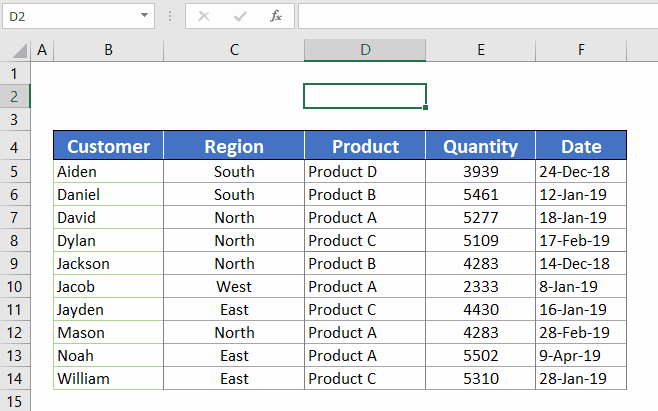## Result

When you enter the formula, the result says Product B. This is supposed to be the value of the cell in the 5th row of the 3rd column, which is cell D9. In other words, the intersection cell of row 5 and column 3.

## More INDEX ExamplesWith this data, try your hands on more examples of the INDEX function to gain some experience. You can download the example file below and practice with.

The main reason I brought these formulas is for you to see the different ways you can refer to ranges when dealing with the INDEX function.

See the second row, for instance, I only specify the array and the row number. I still got a result even though I didn’t specify the column number. This is because the range is one dimensional, meaning it has only one column. Even if you don’t bring the column number, excel can still work with that since it got no options to choose from.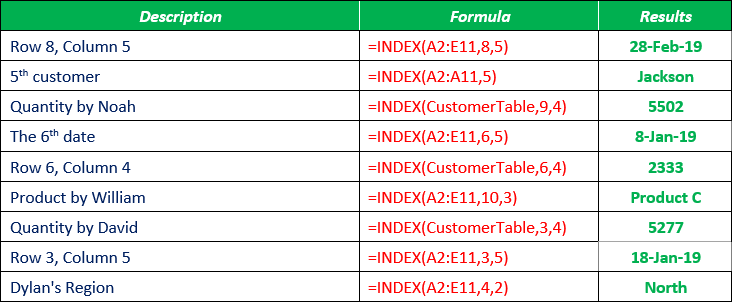Now let’s look at the other version of the INDEX – Reference form.

## Index function syntax (Reference Form)

The array form of the INDEX function has been explained into considerable details. Now let’s focus our attention on the other form of the function – the reference form.

Syntax: INDEX(reference, row_num, [column_num], [area-num])

One thing you should take note is the key difference between the two forms which lies in the choice of arguments for the function. While the Array form of the INDEX works with a single table, the reference form can work with multiple tables. Therefore, you don’t need to use the Reference form of the INDEX function if you are referring to only one table in the formula.

Let’s get familiar with the arguments of this form of INDEX.

• reference: This represents a reference to one or more cell ranges.
• Row_num: This argument specifies the number of a row in a reference (or range of cells). If each range in the reference contains only one row, the row_num argument is optional.
• [Column_num]: Specifies the number of a column in a reference (or range of cells). If each range in the reference contains only one column, then the column_num argument is optional.
• [area_num]: This argument specifies one of the ranges in reference from which to return the intersection cell of a specified row and column. Since the Reference form of the INDEX function works with multiple tables, the [area_num] argument is used to select the table to use. 1 is used to select the first area (or table/range), 2 for the second area (or table/range), and so on.

## INDEX() function example 2 (Reference form)The above picture shows two tables with different data. Our goal here is to use both of these tables as references inside the INDEX function.

Therefore, imagine you want to write a formula that will refer to these two tables and retrieve a value from the second table.

Let’s take, for example, quantity for Daniel is in table two. Daniel is in the 7th row (excluding the header row) whilst quantity is in the 4th column. We then need the formula to retrieve the intersection cell of row 7 and column 4.

Below is the formula:

=INDEX((B6:E15,G6:J15),7,4,2)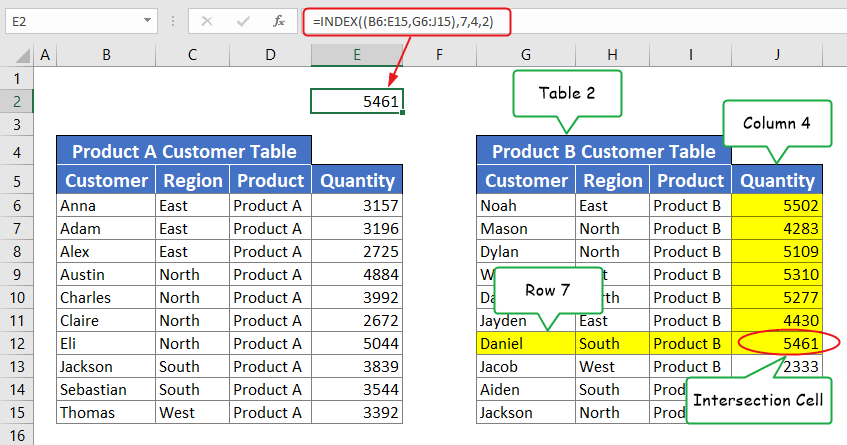## INDEX Formula breakdown

I mentioned before that the main difference between the Array form and Reference form of the INDEX function lies in the arguments. If you have only one table to deal with, go with the array form, otherwise, the reference form is what you need.

Here’s the case we have two tables and want to include both in the INDEX formula. Let’s see how you can do that.

## 1st argument: Reference

This argument is what differentiates between the two forms of INDEX. In the reference version, this argument can refer to more than one table.

To refer to more ranges (or tables) than one, surround the ranges with parenthesis and separate them with a comma.

See screenshot:2nd and 3rd arguments

The 2nd and 3rd arguments determine the row and column numbers respectively. These arguments, in particular, doesn’t change at all as compared to the Array form of the INDEX function. The 7 and 4 specify the row and column numbers respectively. The new thing here is the last argument – [area_num].

Final argument: [area_num]

What the area number argument does is very simple. It specifies which range in the reference to use. Since we are referring to multiple tables in the reference, we need to specify the table we want to work with. An argument of 1 will refer to the first table, argument of 2 to the second table, and so on.

Bonus tips

Writing formulas that include values or text is not a good practice at all. If an argument requires a value or a text, whenever you wish to change this argument, you’ll be forced to crack open the formula for editing.

This is not a problem when you have only one or a few formulas on the line. It only becomes a big problem when you are working with countless formulas.

To avoid these problems in your formulas, always try and avoid using values and use cell references instead.

In other words, if your formula is going to need an argument which is a value (say 2), don’t write the value (2) into the formula. Instead, write the value (2) into another cell and then refer to that cell in the formula. This way, whenever you want to change this particular argument to 4, all you need to do is change the value in that cell.

Let’s try this idea with the first example we worked on.

See screenshot:As seen in the above example, instead of using 5 for the row number argument and 2 for the column number argument, the formula referred to cells C2 and C3 which contain the values to be used for the arguments.

Assuming you want to change the row number or column number, you don’t have to edit the formula to do so. Changing the content in Cells C2 and C3 is all it’ll take.

See screenshot: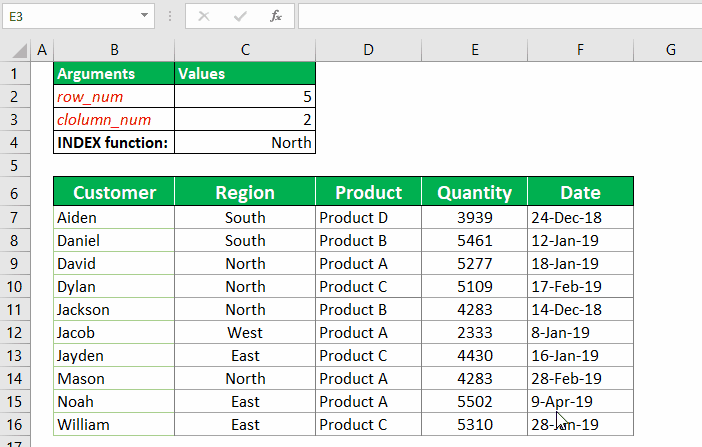As seen in the above example, as you edit the content in cells C2 and C3, the result for the INDEX function also changes.